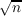#### Vol. 42, No. 3, 1972

 Download this articleFor screen For printingRecent Issues Vol. 325: 1  2 Vol. 324: 1  2 Vol. 323: 1  2 Vol. 322: 1  2 Vol. 321: 1  2 Vol. 320: 1  2 Vol. 319: 1  2 Vol. 318: 1  2Online Archive Volume: Issue:The Journal Subscriptions Editorial Board Officers Contacts Submission Guidelines Submission Form Policies for Authors ISSN: 1945-5844 (e-only) ISSN: 0030-8730 (print) Special Issues Author Index To Appear Other MSP Journals
A probabilistic method for the rate of convergence to the Dirichlet problem

### David F. Fraser

Vol. 42 (1972), No. 3, 657–665
##### Abstract

The expectation Ep(Φ) approximates the solution u(z) = EW(Φ) of the Dirichlet problem for a plane domain D with boundary conditions ϕ on the boundary γ of D, where W is Wiener measure, P is the measure generated by a random walk which approximates Brownian motion beginning at z, and Φ is the functional on paths which equals the value of ϕ at the point where the path first meets γ. This paper develops a specific rate of convergence. If γ is C2, and Pn is generated by random walks beginning at z, with independent increments in the coordinate directions at intervals 1∕n, with mean zero, variance 1, and absolute third moment bounded by M, then |Epn(Φ) EW(Φ)|(CMV∕ρ(z,γ))n116(log n)98, where V is the total variation of ϕ on γ,ρ(z,γ) is the distance from z to γ, and C is a constant depending only on γ.

##### Mathematical Subject Classification 2000
Primary: 60J15
Secondary: 31A25, 65N15
##### Milestones